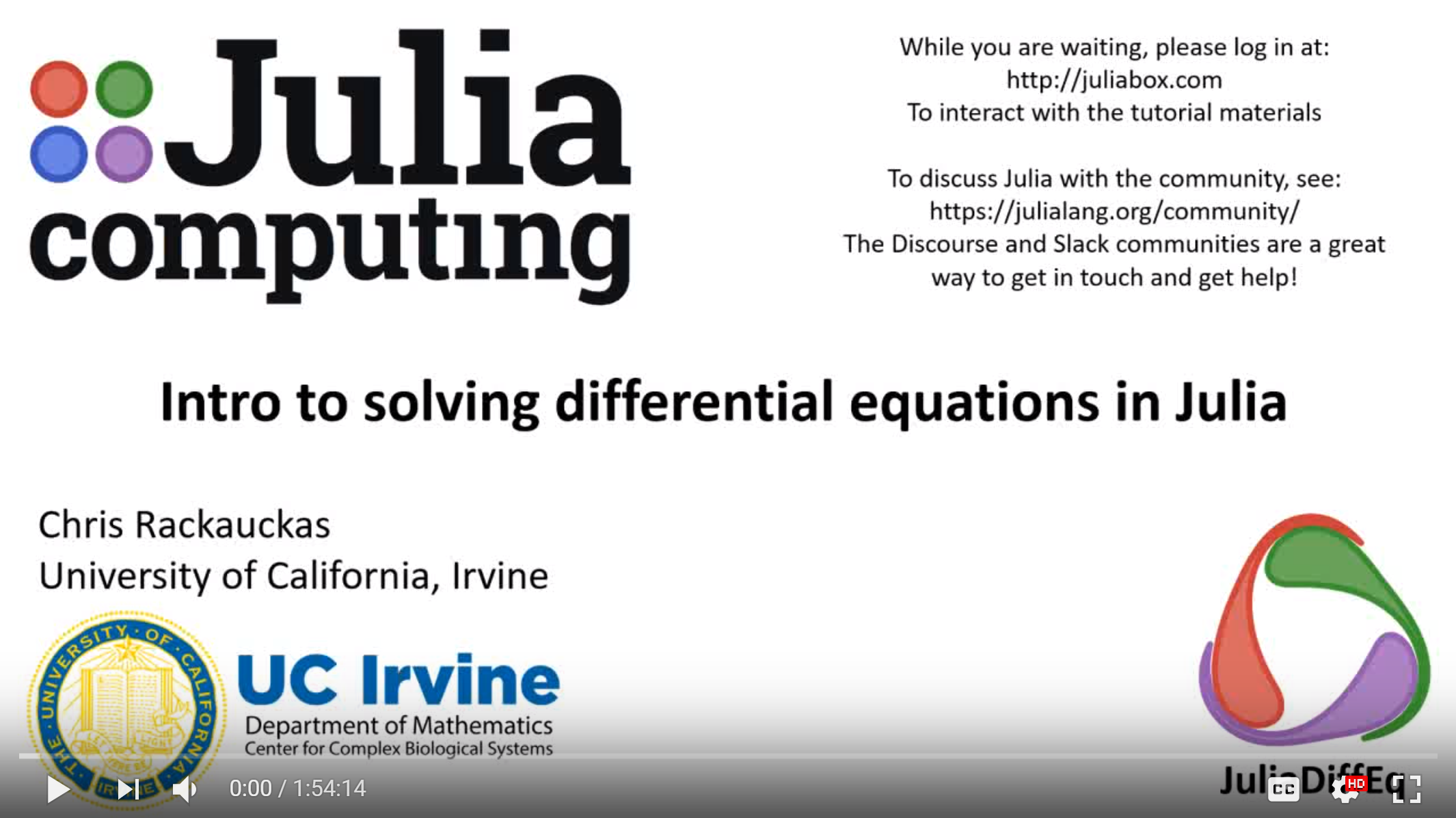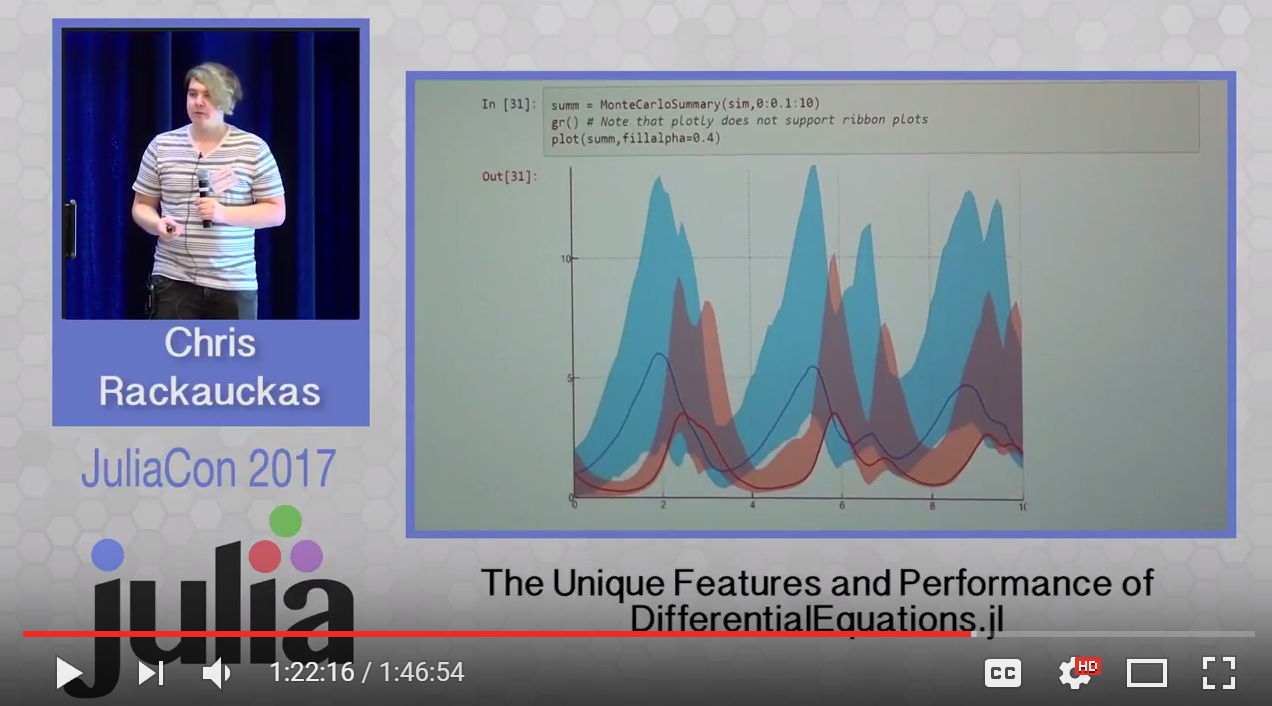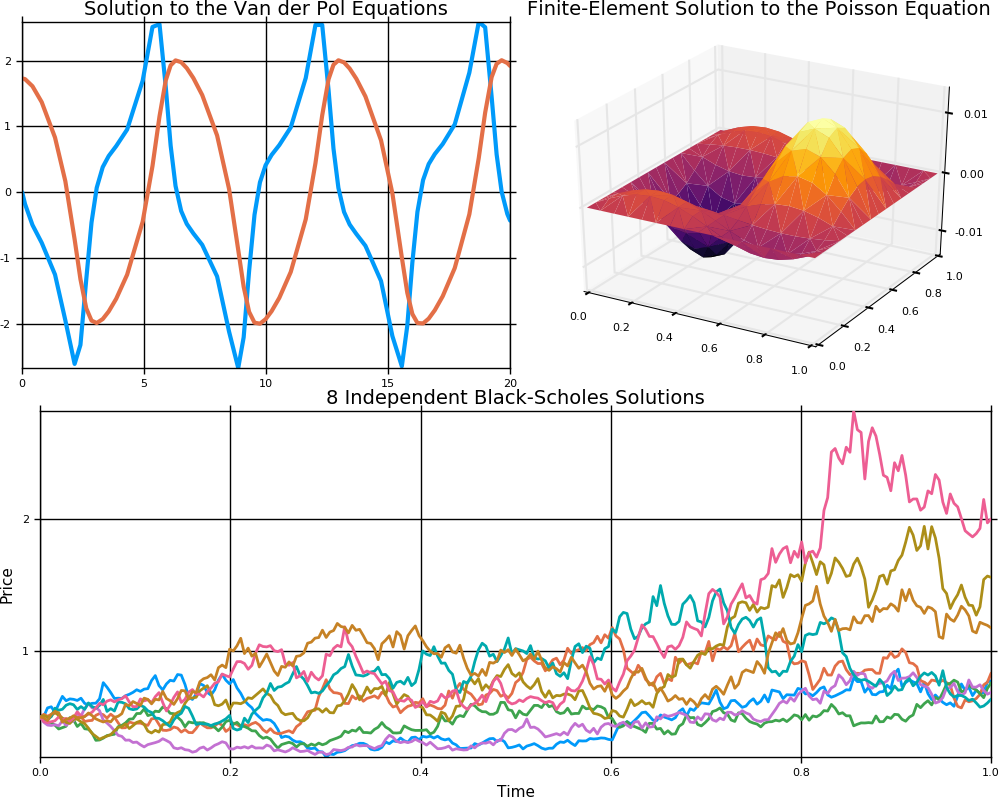Contributors
Last Commit
Mar. 23, 2019
Created
May. 11, 2016

# DifferentialEquations.jl

This is a suite for numerically solving differential equations in Julia. The purpose of this package is to supply efficient Julia implementations of solvers for various differential equations. Equations within the realm of this package include:

• Discrete equations (function maps, discrete stochastic (Gillespie/Markov) simulations)
• Ordinary differential equations (ODEs)
• Split and Partitioned ODEs (Symplectic integrators, IMEX Methods)
• Stochastic ordinary differential equations (SODEs or SDEs)
• Random differential equations (RODEs or RDEs)
• Differential algebraic equations (DAEs)
• Delay differential equations (DDEs)
• Mixed discrete and continuous equations (Hybrid Equations, Jump Diffusions)
• (Stochastic) partial differential equations ((S)PDEs) (with both finite difference and finite element methods)

The well-optimized DifferentialEquations solvers benchmark as the some of the fastest implementations, using classic algorithms and ones from recent research which routinely outperform the "standard" C/Fortran methods, and include algorithms optimized for high-precision and HPC applications. At the same time, it wraps the classic C/Fortran methods, making it easy to switch over to them whenever necessary. It integrates with the Julia package sphere, for example using Juno's progress meter, automatic plotting, built-in interpolations, and wraps other differential equation solvers so that many different methods for solving the equations can be accessed by simply switching a keyword argument. It utilizes Julia's generality to be able to solve problems specified with arbitrary number types (types with units like Unitful, and arbitrary precision numbers like BigFloats and ArbFloats), arbitrary sized arrays (ODEs on matrices), and more. This gives a powerful mixture of speed and productivity features to help you solve and analyze your differential equations faster.

For information on using the package, see the stable documentation. Use the latest documentation for the version of the documentation which contains the un-released features.

All of the algorithms are thoroughly tested to ensure accuracy via convergence tests. The algorithms are continuously tested to show correctness. IJulia tutorial notebooks can be found at DiffEqTutorials.jl. Benchmarks can be found at DiffEqBenchmarks.jl. If you find any equation where there seems to be an error, please open an issue.

If you have any questions, or just want to chat about solvers/using the package, please feel free to chat in the Gitter channel. For bug reports, feature requests, etc., please submit an issue. If you're interested in contributing, please see the Developer Documentation.

## Supporting and Citing

The software in this ecosystem was developed as part of academic research. If you would like to help support it, please star the repository as such metrics may help us secure funding in the future. If you use JuliaDiffEq software as part of your research, teaching, or other activities, we would be grateful if you could cite our work. Please see our citation page for guidelines.

## Video Tutorial## Video Introduction## Comparison to MATLAB, R, Julia, Python, C, Mathematica, Maple, and FortranSee the corresponding blog post

## Example Images